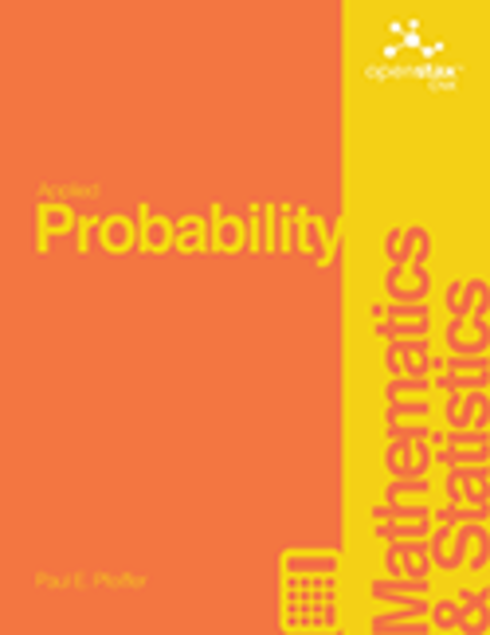# Applied Probability

(0 reviews)

No ratings

Paul Pfeiffer, Rice University

Publisher: OpenStax CNX

Language: English

## Conditions of UseAttribution
CC BY

• 1 Preface
• 2 Probability Systems
• 3 Minterm Analysis
• 4 Conditional Probability
• 5 Independence of Events
• 6 Conditional Independence
• 7 Random Variables and Probabilities
• 8 Distribution and Density Functions
• 9 Random Vectors and joint Distributions
• 10 Independent Classes of Random Variables
• 11 Functions of Random Variables
• 12 Mathematical Expectation
• 13 Variance, Covariance, Linear Regression
• 14 Transform Methods
• 15 Conditional Expectation, Regression
• 16 Random Selection
• 17 Conditional Independence, Given a Random Vector
• 18 Appendices

## Ancillary Material

• Submit ancillary resource

This is a "first course" in the sense that it presumes no previous course in probability. The mathematical prerequisites are ordinary calculus and the elements of matrix algebra. A few standard series and integrals are used, and double integrals are evaluated as iterated integrals. The reader who can evaluate simple integrals can learn quickly from the examples how to deal with the iterated integrals used in the theory of expectation and conditional expectation. Appendix B provides a convenient compendium of mathematical facts used frequently in this work. And the symbolic toolbox, implementing MAPLE, may be used to evaluate integrals, if desired.

In addition to an introduction to the essential features of basic probability in terms of a precise mathematical model, the work describes and employs user defined MATLAB procedures and functions (which we refer to as m-programs, or simply programs) to solve many important problems in basic probability. This should make the work useful as a stand-alone exposition as well as a supplement to any of several current textbooks.

Most of the programs developed here were written in earlier versions of MATLAB, but have been revised slightly to make them quite compatible with MATLAB 7. In a few cases, alternate implementations are available in the Statistics Toolbox, but are implemented here directly from the basic MATLAB program, so that students need only that program (and the symbolic mathematics toolbox, if they desire its aid in evaluating integrals).

Since machine methods require precise formulation of problems in appropriate mathematical form, it is necessary to provide some supplementary analytical material, principally the so-called minterm analysis. This material is not only important for computational purposes, but is also useful in displaying some of the structure of the relationships among events.